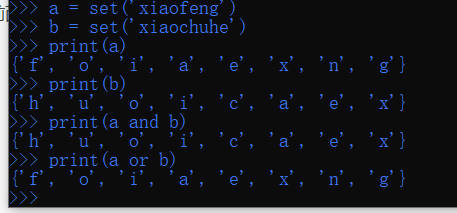# 一、概述

python中的逻辑操作符and 和or，也叫惰性求值，由于是惰性，只要确定了值就不往后解析代码了。

# 二、用法说明

## （一）and 用法

``````print (1 > 2 and 0 < 1)#False
print (1 > 2 and 0 > 1) #False
print (1 < 2 and 0 < 1)#True
print (1 > 2 and 0 < 1)#False
print (1 > 2 and 0 < 1 and 2 > 0 and 5 > 1) #False``````

``````print (0 and 5) #0
print (1 and 5) #5
print (5 and 0) #0
print (10 and 0 and 5 and 0)#0
print (6 and 4 and 3)#3
print (1 and 4 and 2 and 6) #6``````

## （二）or 用法

``````print (1 > 2 or 0 < 1) #True
print (1 < 2 or 0 > 1) #True
print (1 > 2 or 0 > 1) #False
print (1 < 2 or 0 > 1 or 2 > 3)#True``````

``````print (0 or 10) #10
print (1 or 2)  #1
print (1 or 0)  #1
print (7 or 5 or 5)#7
print (9 or 5 or 6 or 0 or 8)#9``````

# 三、两个集合and 和or操作的时候的问题``````print (1 and 5 or 0) #先计算1 and 5 为5再计算5 or 0 为5
print (1 or 5 and 0 or 6 and 0)
'''

'''``````